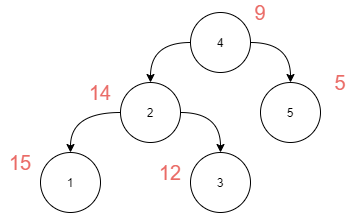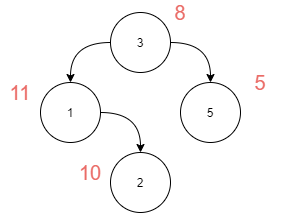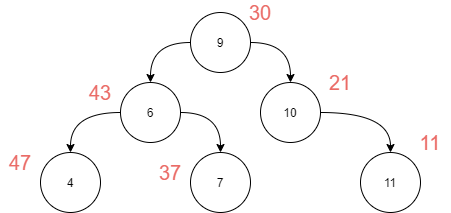1

# BST to greater tree

Difficulty: MEDIUMContributed By
Sounak Majumder
Avg. time to solve
25 min
Success Rate
65%

Problem Statement

#### A binary search tree (BST) is a binary tree data structure that has the following properties.

``````• The left subtree of a node contains only nodes with data less than the node’s data.

• The right subtree of a node contains only nodes with data greater than the node’s data.

• Both the left and right subtrees must also be binary search trees.
``````
##### Example:``````Because only 5 is greater than 4 in the above BST, node 4 will be updated to 9 (4 + 5 = 9).

Nodes 3, 4, and 5 are greater than 2, hence node 2 will be modified to 14 (2 + 3 + 4 + 5 = 14).

Node with data 5 will remain the same because there is no node in the BST with data greater than 5.

Nodes 2,3, 4, and 5 are greater than 1, hence node 1 will be modified to 15 (1 + 2 + 3 + 4 + 5 = 15).

Nodes 4 and 5 are greater than 3, hence node 3 will be modified to 12 (3 + 4 + 5 = 12).
``````
##### Input Format:
``````The first line contains an Integer 'T' which denotes the number of test cases or queries to be run. Then the test cases follow.

The first line of input contains the elements of the tree in the level order form separated by a single space.

If any node does not have a left or right child, take -1 in its place. Refer to the example below.

Example:

Elements are in the level order form. The input consists of values of nodes separated by a single space in a single line. In case a node is null, we take -1 in its place.

For example, the input for the tree depicted in the below image would be :
````````````1
2 3
4 -1 5 6
-1 7 -1 -1 -1 -1
-1 -1

Explanation :
Level 1 :
The root node of the tree is 1

Level 2 :
Left child of 1 = 2
Right child of 1 = 3

Level 3 :
Left child of 2 = 4
Right child of 2 = null (-1)
Left child of 3 = 5
Right child of 3 = 6

Level 4 :
Left child of 4 = null (-1)
Right child of 4 = 7
Left child of 5 = null (-1)
Right child of 5 = null (-1)
Left child of 6 = null (-1)
Right child of 6 = null (-1)

Level 5 :
Left child of 7 = null (-1)
Right child of 7 = null (-1)

The first not-null node (of the previous level) is treated as the parent of the first two nodes of the current level. The second not-null node (of the previous level) is treated as the parent node for the next two nodes of the current level and so on.

The input ends when all nodes at the last level are null (-1).
``````
##### Note :
``````The above format was just to provide clarity on how the input is formed for a given tree.

The sequence will be put together in a single line separated by a single space. Hence, for the above-depicted tree, the input will be given as:

1 2 3 4 -1 5 6 -1 7 -1 -1 -1 -1 -1 -1
``````
##### Output Format :
``````For each test case, print a single line containing a single integer denoting the root node of the modified BST.

The output for every test case will be denoted in a separate line.
``````
##### Note:
``````You are not required to print anything explicitly. It has already been taken care of. Just implement the function.
``````
##### Constraint :
``````1 <= T <= 100
0 <= N <= 3000
-10 ^ 6 <= DATA <= 10 ^ 6

Where 'N' is the number of nodes in the tree, 'T' represents the number of test cases and 'DATA' denotes data contained in the node of the binary tree.

Duplicate elements can be in the right subtree or left subtree.

Time Limit: 1 sec.
``````
##### Sample Input 1:
``````2
3 1 5 -1 2 -1 -1 -1 -1
9 6 10 4 7 -1 11 -1 -1 -1 -1 -1 -1
``````
##### Sample Output 1:
`````` 8 11 5 -1 10 -1 -1  -1 -1
30 43 21 47 37 -1 11 -1 -1 -1 -1 -1 -1
``````
##### Explanation of the Sample Input1:
``````Here we have 2 test cases, hence there are 2 binary trees

Test Case 1:
````````````Node 5 is greater than node 3, hence node 3 modifies to 8 (5 + 3 = 8).

Nodes 2,3 and 5 are greater than node 1, hence node 1 modifies to 10 (1 + 5 + 3 + 2 = 11).

No node is greater than node 5, hence node 5 remains unchanged.

Nodes 3 and 5 are greater than node 2, hence node 2 modifies to 10 (5 + 3 + 2 = 10).

Test Case 2:
````````````Nodes 10 and 11 are greater than node 9, hence node 9 modifies to 30 (10 + 11 + 9 = 30).

Nodes 7,9,11 and 10 are greater than node 6, hence node 6 modifies to 43 (7 + 9 + 11 + 10 + 6 = 43).

Node 11 is greater than node 10, hence node 10 modifies to 21 (10 + 11 = 21).

Nodes 6,7,9,11 and 10 are greater than node 4, hence node 4 modifies to 47 (7 + 9 + 11 + 10 + 6 + 4 = 47).

Nodes 9,10 and 11 are greater than node 7, hence node 7 modifies to 37 (10 + 11 + 9 + 7 = 37).

No node is greater than node 11, hence node 11 remains unchanged.
``````Console